Processing ......FreeComputerBooks.com Links to Free Computer, Mathematics, Technical Books all over the World

Category Theory
Related Book Categories:
•An Invitation to Applied Category Theory: Seven Sketches

Category theory is now a powerful tool in science, informatics, and industry. This book offers a self-contained tour of applied category theory. Each chapter follows a single thread motivated by a real-world application and discussed with category-theoretic tools.

•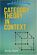Category Theory in Context (Emily Riehl)

This book introduces the essential concepts of category theory: categories, functors, natural transformations, the Yoneda lemma, limits and colimits, adjunctions, monads, Kan extensions, and other topics.

•Topology: A Categorical Approach (Tai-Danae Bradley, et al)

A graduate-level textbook that presents basic topology from the perspective of category theory. Many graduate students are familiar with the ideas of point-set topology and they are ready to learn something new about them.

•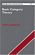Basic Category Theory (Tom Leinster)

Assuming little mathematical background, this short introduction to Category Theory is ideal for beginning graduate students or advanced undergraduates learning category theory for the first time.

•Category Theory for the Sciences (David I. Spivak)

Using databases as an entry to Category Theory, this book explains category theory by examples, and shows that category theory can be useful outside of mathematics as a rigorous, flexible, and coherent modeling language throughout the sciences.

•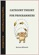Category Theory for Programmers (Bartosz Milewski)

In this category theory for programmers, the author illustrates all major concepts using computer code. You are probably aware that functional languages are closer to math than the more popular imperative languages. They also offer more abstracting power.

•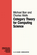Category Theory for Computing Science (Michael Barr, et al.)

This book is a textbook in basic category theory, written specifically to be read by people in computing science. It expounds the constructions we feel are basic to category theory in the context of examples and applications to computing science.

•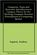Categories, Types, and Structures (Andrea Asperti, et al)

This book introduces Category Theory at a level appropriate for computer scientists and provides practical examples in the context of programming language design. It pursues the more complex mathematical semantics of data types and programs.

•Categorical Homotopy Theory (Emily Riehl)

This book develops abstract homotopy theory from the categorical perspective with a particular focus on examples. It helps consolidate and simplify one's understanding of derived functors, homotopy limits and colimits, and model categories, among others.

•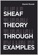Sheaf Theory through Examples (Daniel Rosiak)

Taking an applied category theory perspective, this book provides an approachable introduction to elementary sheaf theory and examines applications. It seeks to bridge the powerful results of sheaf theory as used by mathematicians and real-world applications,

•Higher Topos Theory (Jacob Lurie)

This book presents the foundations of Higher Topos Theory, using the language of weak Kan complexes, and shows how existing theorems in algebraic topology can be reformulated and generalized in the theory's new language.

•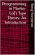Programming in Martin-Lof's Type Theory: An Introduction

This book focuses on the type theory developed by Per Martin-Lof. It contains a thorough introduction to the Martin-Lof's Type Theory, with information on polymorphic sets, subsets, monomorphic sets, and a full set of helpful examples.

Book Categories
 :All CategoriesTop BooksRecent BooksMiscellaneous BooksComputer LanguagesComputer ScienceData Science/DatabasesElectrical EngineeringJava and Java EE (J2EE)Linux and UnixMathematicsMicrosoft and .NETMobile ComputingNetworking and CommunicationsSoftware EngineeringSpecial TopicsWeb Programming
Other Categories